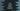# C++ cmath isless function explanation with example## C++ cmath isless function:

isless function is defined in the cmath header file. It takes two numbers as the parameters and checks if one is less than the other.

In this post, we will learn how to use isless function with examples.

### Definition of isless function:

isless function is defined as like below (C++11):

``````bool isless (float x, float y);
bool isless (double x, double y);
bool isless (long double x, long double y);``````

#### Parameters and return value of isless:

As you can see here, it takes two parameters and returns one boolean value. It returns whether x is less than y. If yes, it returns true. Else it returns false.

If one or both members are NaN, it returns false.

### Example of isless:

Let’s take an example of isless:

``````#include <iostream>
#include <cmath>

int main ()
{

std::cout<<std::isless(5, 6)<<'\n';
std::cout<<std::isless(50.6, 16)<<'\n';
std::cout<<std::isless(51.22, 62.33)<<'\n';

return 0;
}``````

If you run this program, it will print:

``````1
0
1``````

The second statement returns false or 0. Other statements are returning true.